MODEL Statement

<label:>
MODEL response = <effects> </ options> ;

Main effects and interaction terms can be specified in the MODEL statement, as in the GLM procedure ( Chapter 41, The GLM Procedure. )

The optional label, which must be a valid SAS name, is used to label the model in the OUTEST data set.

You can specify the following options for the model fit.

ALPHA=value

specifies the significance level for the confidence intervals for regression parameters. The value must be between 0 and 1. By default, ALPHA=0.05.

CORRB

produces the estimated correlation matrix of the parameter estimates.

COVB

produces the estimated covariance matrix of the parameter estimates.

CUTOFF=value

specifies the multiplier of the cutoff value for outlier detection. By default, CUTOFF=3.

DIAGNOSTICS<(ALL)>

requests the outlier diagnostics. By default, only observations identified as outliers or leverage points are displayed. To request that all observations be displayed, specify the ALL option.

FAILRATIO=value

specifies the threshold of failure ratio for the subsampling algorithm of an LTS or S estimate. It also applies to the initial LTS or S step in an MM estimate. The threshold must be between 0 and 1. Its default value is 0.99. See the section LTS Estimate or S Estimate for details.

ITPRINT

displays the iteration history for the iteratively reweighted least squares algorithm used by M and MM estimation. You can also use this option in the PROC statement.

LEVERAGE <(leverage-options)>

requests an analysis of leverage points for the covariates. The results are added to the diagnostics table, which you can request with the DIAGNOSTICS option in the MODEL statement.

The following leverage-options are available:

CUTOFF=value

specifies the leverage cutoff value for leverage-point detection. See the section Leverage Point and Outlier Detection for details. The cutoff value can also be specified with the leverage cutoffvalue by using the CUTOFFALPHA= option.

CUTOFFALPHA=value

specifies the leverage cutoffvalue for leverage-point detection. The respective leverage cutoff value equals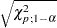(or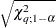if projection is applied in the generalized MCD algorithm). By default,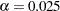.

H | QUANTILE=n

specifies the quantile to be minimized for the MCD algorithm that is used for the leverage-point analysis. By default, H=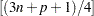, where n is the number of observations and p is the number of independent variables excluding the intercept.

MCDALPHA=value

specifies the MCD cutoffvalue for the final MCD reweighting step. The respective MCD cutoff value equals(orif projection is applied in the generalized MCD algorithm). By default,.

MCDCUTOFF | MCDCUTOFF=value

specifies the MCD cutoff value for the final MCD reweighting step. See the section Mahalanobis Distance versus Robust Distance and Rousseeuw and Van Driessen (1999) for details. The cutoff value can also be specified with the MCD cutoffvalue by using the MCDALPHA= option.

MCDINFO

requests that detailed information about the MCD covariance estimate be displayed, including the low-dimensional structure, the breakdown value, the MCD center, and the MCD covariance itself. The option outputs the ODS tables of the MCD profile, MCD center, MCD covariance, and MCD correlation.

OPC | OFFPLANECOEF

requests the ODS table of the coefficients for MCD-dropped components, when projection is applied in the generalized MCD algorithm. The OFFPLANECOEF option is ignored for the regular MCD algorithm.

PALPHA | PROJECTIONALPHA=value

specifies the projection cutoffvalue to be used to judge whether an observation is on or off the low-dimensional hyperplane identified by the generalized MCD algorithm. The respective projection cutoff value equals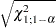. By default,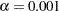.

PCUTOFF | PROJECTIONCUTOFF=value

specifies the projection cutoff value to be used to judge whether an observation is on or off the low-dimensional hyperplane identified by the projected MCD algorithm. See the section Mahalanobis Distance versus Robust Distance and Rousseeuw and Van Driessen (1999) for details. The projection cutoff value can also be specified with the projection cutoffvalue by using the PALPHA= option.

PTOL | PROJECTIONTOLERANCE=value

specifies the projection tolerance value for the low-dimensional structure detection. See the section Leverage Point and Outlier Detection for details.

NOGOODFIT

suppresses the computation of goodness-of-fit statistics.

NOINT

specifies no-intercept regression.

SINGULAR=value

specifies the tolerance for testing singularity of the information matrix and the crossproducts matrix for the initial least squares estimates. Roughly, the test requires that a pivot be at least value times the original diagonal value. By default, SINGULAR=1.E12.